Students can Download Computer Science Chapter 12 Arrays and Structures Questions and Answers, Notes Pdf, Samacheer Kalvi 11th Computer Science Book Solutions Guide Pdf helps you to revise the complete Tamilnadu State Board New Syllabus and score more marks in your examinations.

## Tamilnadu Samacheer Kalvi 11th Computer Science Solutions Chapter 12 Arrays and Structures

### Samacheer Kalvi 11th Computer Science Arrays and Structures Text Book Back Questions and Answers

PART – 1
I. Choose The Correct Answer

Question 1.
Which of the following is the collection of variables of the same type that an referenced by a common name?
(a) int
(b) float
(c) Array
(d) class
Answer:
(c) Array

Question 2.
Array subscripts always starts with which number?
(a) – 1
(b) 0
(c) 2
(d) 3
Answer:
(b) 0Question 3.
int age[ ]={6, 90, 20, 18, 2}; How many elements are there in this array?
(a) 2
(b) 5
(c) 6
(d) 4
Answer:
(b) 5

Question 4.
cin >> n; To which element does this statement accepts the value?
(a) 2
(b) 3
(c) 4
(d) 5
Answer:
(c) 4

Question 5.
By default, a string ends with which character?
(a) \o
(b) \t
(c) \n
(d) \b
Answer:
(a) \o

PART – 2
II. Answers to all the questions

Question 1.
What is Traversal in an Array?
Answer:
Accessing each element of an array at least once to perform any operation is known as “Traversal”. Displaying all the elements in an array is an example of “traversal”.

Question 2.
What is Strings?
Answer:
A string is defined as a sequence of characters where each character may be a letter, number or a symbol. Each element occupies one byte of memory. Every string is terminated by a null (‘\0’, ASCII code 0) character which must be appended at the end of the string.Question 3.
What is the syntax to declare two – dimensional array.
Answer:
The declaration of a 2 – D array is
data – type array_name[row – size] [col – size];
In the above declaration, data-type refers to any valid C++ data – type, array _ name refers to the name of the 2 – D array, row – size refers to the number of rows and col-size refers to the number of columns in the 2 – D array.

PART – 3
III. Answers to all the questions

Question 1.
Define an Array. What are the types?
Answer:
“An array is a collection of variables of the same type that are referenced by a common name”. An array is also a derived datatype in C++.
There are different types of arrays used in C++. They are:

1. One – dimensional arrays
2. Two – dimensional arrays
3. Multi – dimensional arrays

Question 2.
Write a note on Array of strings.
Answer:
An array of strings is a two – dimensional character array. The size of the first Index (rows) denotes the number of strings and the size of the second index (columns) denotes the maximum length of each string. Usually, array of strings are declared in such a way to accommodate the null character at the end of each string. For example, the 2 – D array has the declaration:
char name ;
In the above declaration,
No. of rows = 7;
No. of columns =10;
We can store 7 strings each of maximum length 10 characters.Question 3.
Write a C++ program to accept and print your name.
Answer:
#include
using namespace std;
int main()
{
charname;
cout<< “Enter your name:”; cin >>name;
cout<< “My name is”<< name;
}
Output:
Enter your name: PRIYA
My name is PRIYA

PART – 4
IV. Answers to all the questions

Question 1.
Write a C++ program to find the difference between two matrix.
Answer: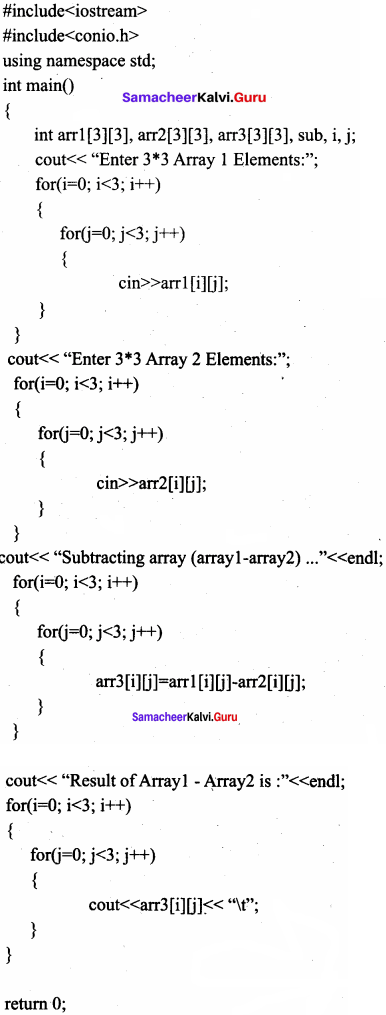Output
Enter 3*3 Array 1 Elements :
10 11 12
13 14 15
16 17 18

Enter 3*3 Array 2 Elements :
123
456
789

Subtracting array (array1 – array2)
Result of Array 1 – Array2 is :
9 9 9
9 9 9
9 9 9

Question 2.
How will you pass two dimensional array to a function explain with example.
Answer:
Passing 2”D array to a function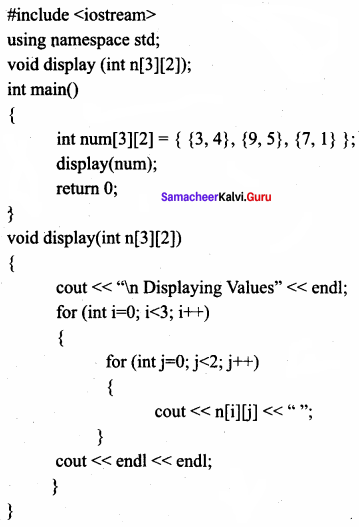Output:
Displaying Values
3 4
9 5
7 1

PART – 1
I. Choose the correct answer

Question 1.
The data elements in the structure are also known as ………………..
(a) objects
(b) members
(c) data
(d) records
Answer:
(a) objects

Question 2.
Structure definition is terminated by
(a) :
(b) }
(c) ;
(d) ::
Answer:
(c) ;Question 3.
What will happen when the structure is declared?
(a) it will not allocate any memory
(b) it will allocate the memory
(c) it will be declared and initialized
(d) it will be only declared
Answer:
(b) it will allocate the memory

Question 4.
What is the output of this program?
Answer:
#include
#include
using namespace std;
int main()
{

struct student
{
int n;
char name;
};
student s;
s.n = 123;
strcpy(s.name, “Balu”);
cout <<s.n;
cout<< s.name << endl;

return 0; }
(a) 123Balu
(b) BaluBalu
(c) Balul23
(d) 123 Balu
Answer:
(d) 123 Balu

Question 5.
A structure declaration is given below,
struct Time
{
int hours;
int minutes;
int seconds;
} t;
Using above declaration which of the following refers to seconds.
(a) Time.seconds
(b) Time::seconds
(c) seconds
(d) t. seconds
Answer:
(d) t. seconds

Question 6.
What will be the output of this program?
#include
using namespace std;
struct Shoe Type
{
string name;
double price;
};
int main()
{
ShoeType shoel, shoe2;
shoe 1.name = “Adidas”;
shoel.price = 9.99;
cout << shoel.name << “#” << shoel.price<<endl;
shoe2 – shoel;
shoe2.price = shoe2.price / 9;
cout << shoe2.name << “#”<< shoe2.price;
return 0;
(a) Adidas # 9.99; Adidas # 1.11
(b) Adidas # 9.99; Adidas # 9.11
(c) Adidas # 9.99; Adidas # 11.11
(d) Adidas # 9.11; Adidas # 11.11
Answer:
(a) Adidas # 9.99; Adidas # 1.11Question 7.
Which of the following is a properly defined structure?
(a) struct {int num;}
(b) struct sum {int num;}
(c) struct sum int sum;
(d) struct sum {int num;};
Answer:
(d) struct sum {int num;};

Question 8.
A structure declaration is given below,
struct employee
{
int empno;
char ename;
} e;
Using above declaration which of the following statement is correct?
(a) cout << e.empno << e.ename;
(b) cout << e.empno << ename;
(c) cout << e->empno << e → ename;
(d) cout << e.empno << e.ename;
Answer:
(a) cout << e.empno << e.ename;

Question 9.
Which of the following cannot be a structure member?
(a) Another structure
(b) Function
(c) Array
(d) variable of double datatype
Answer:
(b) Function

Question 10.
When accessing a structure member, the identifier to the left of the dot operator is the name of …………………
(a) structure variable
(b) structure tag
(c) structure member
(d) structure function
Answer:
(c) structure member

PART – 2
II. Answer to all the questions

Question 1.
Define structure. What is its use?
Answer:
Structure is a user – defined which has the combination of data items with different data types. This allows to group of variables of mixed data types together into a single unit. The structure provides a facility to store different data types as a part of the same logical element in one memory chunk adjacent to each other.

Question 2.
To store 100 integer number which of the following is good to use?
Array or Structure. State the reason.
Answer:
Array is good to use.
Reasons:

1. All 100 numbers are integer type.
2. Array index helps to access the numbers quickly.Question 3.
What is the error in the following structure definition?
struct employee {inteno ;chamame  ;char dept;}
Employee e1,e2;
Answer:
Errors:

1. ‘i’ is missing.
2. Spaces are missing at two places
3. Structure name given wrongly.

Corrected structure:
struct employee {int eno; char ename ;

char dept;}
employee e, e2;

Question 4.
Write a structure definition for the structure student containing examno, name and an array for storing five subject marks.
Answer:
struct student
{
long examno;
char name ;
int marks ;
};Question 5.
Why for pacing a structure to a function call by reference is advisable to us?
Answer:
In this method of passing the structures to functions, the address of a structure variable /object is passed to the function using address of(&) operator. So any change made to the contents of structure variable inside the function are reflected back to the calling function.

PART – 3
III. Answer to all the questions

Question 1.
How will you pass a structure to a function?
Answer:
A structure variable can be passed to a function in a similar way of passing any argument that is of built – in data type.
If the structure itself is an argument, then it is called “call by value”. If the reference of the structure is passed as an argument then it is called, “call by reference”.Question 2.
The following code sums up the total of all students name starting with ‘S’ and display it. Fill in the blanks with required statements.
Answer:
struct student {int exam no, lang, eng, phy, che, mat, csc, total; char name;};
int main()
{
student s;
for(int i = 0;i<20;i++)
{
………………….. //accept student details
}
for(int i=0;i<20;i++)
{
………………….. //check for name starts with letter “S”
………………….. // display the detail of the checked name
}
return 0;
}
Answer: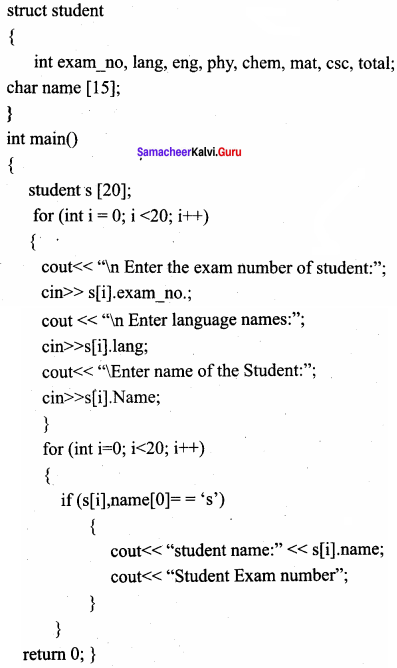Question 3.
How to access members of a structure? Give example.
Answer:
Once objects of a structure type are declared, their members can be accessed directly. The syntax for that is using a dot(.) between the object name and the member name.
Example: student.name;
if the members are a pointer type, then is used to access the members.
Let name be a character pointer in student like char*name
It can be accessed student → name,
struct
{

long rollno;
int age;
float weight;
student;

}
The student can be referred as reference name to the above structure and the elements can be accessed like student.roll no, student.age, and student.weight.

Question 4.
Write the syntax and an example for structure.
Answer:
Structure is declared using the keyword ‘struct’. The syntax of creating a structure is given below.
struct structure_name {

type member_name 1;
type member_name2;

} reference_name;
An optional field reference_name can be used to declare objects of the structure type directly.
Example:
struct Student
{

long rollno;
int age;
float weight;

};Question 5.
What is called anonymous structure? Give an example.
Answer:
A structure without a name/tag is called anonymous structure.
Example:
struct
{
long rollno;
int age;
float weight;
};
The student can be referred as reference name to the above structure and the elements can be accessed like student.rollno, student.age and student.weight.

PART – 4
IV. Answer to all the questions

Question 1.
Explain array of structures with example.
Answer:
A class may contain many students. So, the definition of structure for one student can also be extended to all the students. If the class has 20 students, then 20 individual structures are required. For this purpose, an array of structures can be used. An array of structures is declared in the same way as declaring an array with built – in data types like int or char.
Program that reads the details of 20 students and prints the same.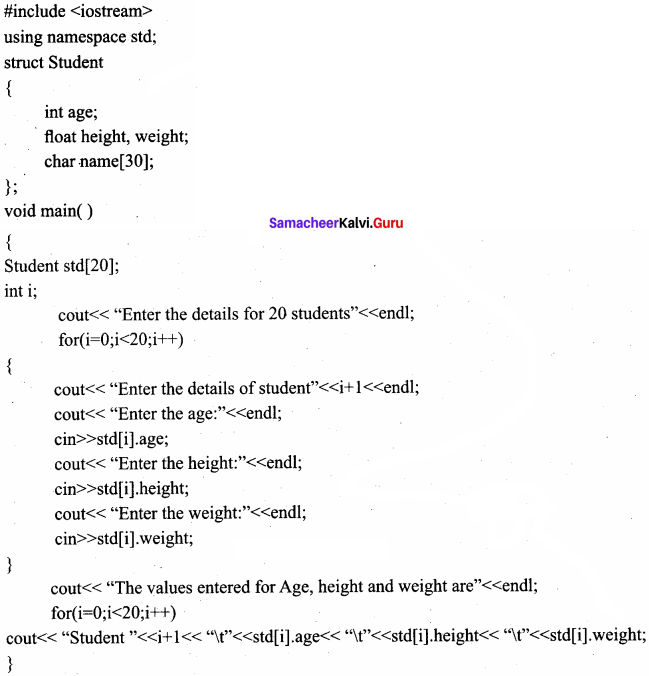Output:
Enter the details of 20 students
Enter the details for student 1

Enter the age:
18
Enter the height:
160.5
Enter the weight:
46.5
Enter the details for student2
Enter the age:
18

Question 2.
Explain call by value with respect to structure.
Answer:
When a structure is passed as argument to a function using call by value method, any change made to the contents of the structure variable inside the function to which it is passed do not affect the structure variable used as an argument.
#include
using namespace std;
struct Employee
{
char name;
int age;
float salary;
};
void printData(Employee); // Function declaration
int main()
{

Employee p;
cout << “Enter Full name:”; cin >> p.name;
cout << “Enter age:”; cin >> p.age;
cout << “Enter salary:”; cin >> p.salary;
// Function call with structure variable as an argument
printData(p);
return 0;

}
void printData(Employee q)
{

cout << “\nDisplaying Information.” << endl;
cout << “Name:” << q.name << endl;
cout <<”Age:” << q.age << endl;
cout << “Salary:” << q.salary;

}

Output:
Enter Full name: Kumar
Enter age : 55
Enter salary : 34233.4
Displaying Information.
Name : Kumar
Age : 55
Salary : 34233.4
In the above example, a structure named Employee is declared and used. The values that are entered into the structure are name, age and salary of a Employee are displayed using a function named printData(). The argument for the above function is the structure Employee. The input can be received through a function named readData().Question 3.
Write a C++ program to add two distances using the following structure definition, struct Distance!
int feet;
float inch;
}d1, d2, sum;
Answer:
int main()
{

cout << “Enter 1st distance:” << end1;
cout << “Enter feet:”; cin >> d1.feet;
cout << “Enter inch:”; cin >> d1.inch;
cout << “\n information for 2nd distance:” << end1;
cout << “Enter feet:”; cin >> d2.feet;
cout << “Enter inch:”; cin >> d2.inch;
sum.feet = d1 . feet + d2.feet;
sum.inch = d1.inch + d2.inch;
if (sum.inch > 12)
{
++ sum.feet;
sum.inch = 12;
}
cout << end1; “Sum of distance =” << sum.feet << “feet” << sum.inch << “inches”;
return 0;

}

Output:
Enter 1 st distance
Enter feet: 6
Enter inch: 3.4
Enter 2nd distance
Enter feet: 5
Enter inch: 10.2
Sum of distances = 12 feet 1.6 inches

Question 4.
Write a C++ program to declare and accept an array of professors. Display the details of the department= “COMP.SCI” and the name of the professors start with ‘A’. The structure “college” should contain the following members.
prof id as integer
name and Department as character array
Answer: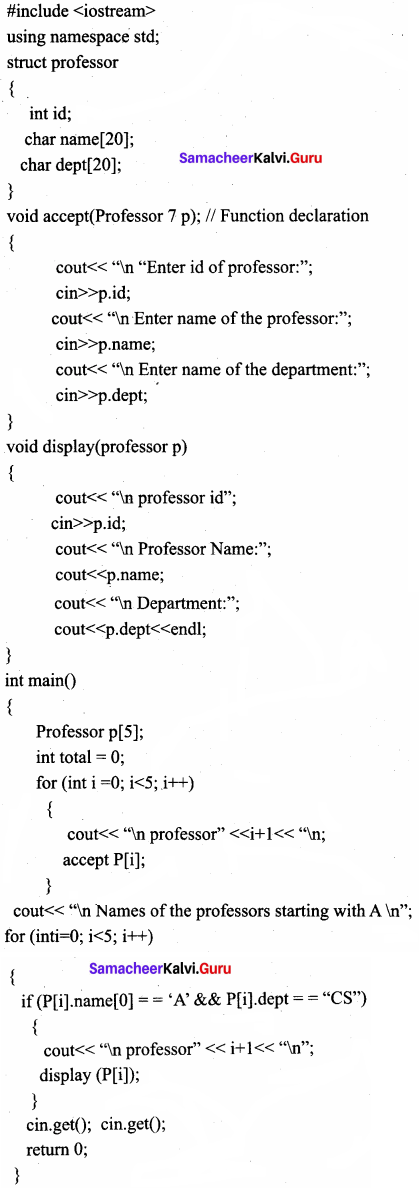Output
Professor 1
Enter id of professor: 100
Enter name of the professor: John
Enter name of the department: CS

Professor 2
Enter id of professor: 101
Enter name of the professor: Janardhan
Enter name of the department: ECE

Professor 3
Enter id of professor: 102
Enter name of the professor: Albert
Enter name of the department: CS

Professor 4
Enter id of professor: 103
Enter name of the professor: Asha
Enter name of the department: CS

Professor 5
Enter id of professor: 104
Enter name of the professor: Han
Enter name of the department: EEE

Name of the professor starting with A
Professor 1
Enter id of professor: 102
Enter name of the professor: Albert
Enter name of the department: CS

Professor 2.
Enter id of professor: 103
Enter name of the professor: Asha
Enter name of the department: CSQuestion 5.
Write the output of the following C++ program
Answer:
#include
#include
#include
#include
using namespace std;
struct books {
char name, author;
}a;
int main()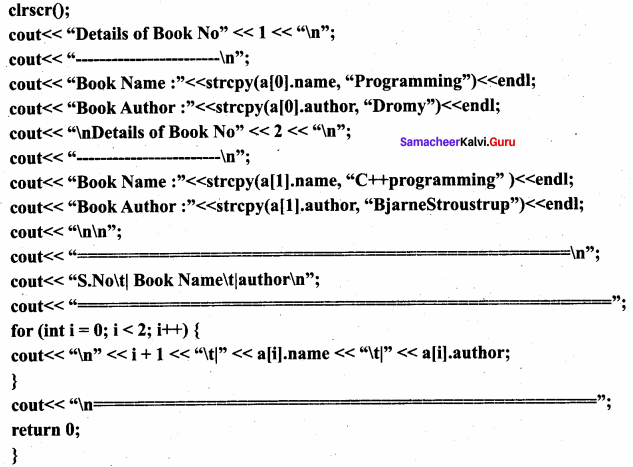Output:
Details of Book No. 1
Book name : Programming
Book author : Dromy
Details of Book No. 2
Book Name : C++ Programming
Book Author : Bjame Stroustrup
1 Iprogrammingl Dromy
2| C++ Programming | Bjame Stroustmp

Question 6.
Write the output of the following C++ program
Answer: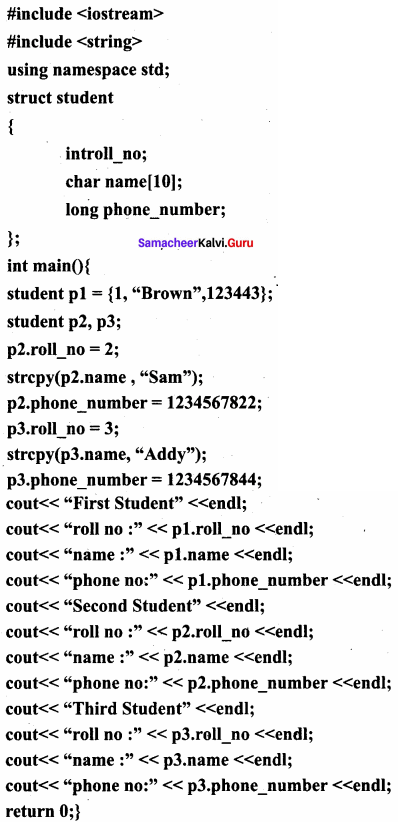Output
First student
roll no : 1
name : Brown
Phone no. : 123443
Second Student
roll no : 2
name : Sam
Phone no. : 1234567
Third Student
roll no : 3
name : Addy
Phone no. : 1234597844

Question 7.
Debug the error in the following program
Answer: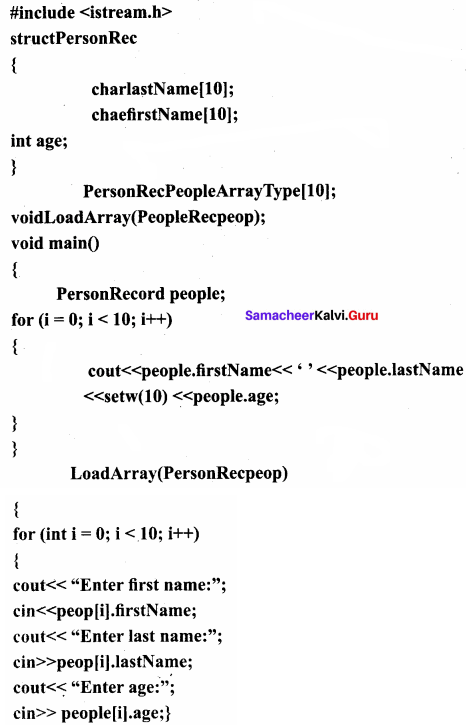Corrected Program: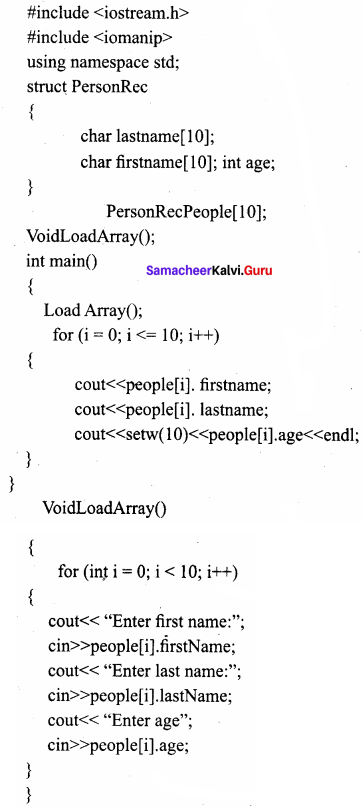### Samacheer kalvi 11th Computer Science Arrays and Structures Additional Questions and Answers

PART – 1
I. Choose the correct answer

Question 1.
The size of the array is referred to as its ………………..
(a) dimension
(b) direction
(c) location
(d) space
Answer:
(a) dimension

Question 2.
The subscript in bracket can be a variable, a constant or an expression to ………………..
(a) character
(b) integer
(c) long double
(d) float
Answer:
(b) integerQuestion 3.
Displaying all the elements in an array is an example of ………………..
(a) memory allocation
(b) call by reference
(c) traversal
(d) none of these
Answer:
(c) traversal

Question 4.
Syntax of character array declaration is ………………..
(a) int array char name [size];
(b) char array [ ];
(c) char_name[size];
(d) char array – name[size];
Answer:
(d) char array – name[size];

Question 5.
During ……………….. the array of elements cannot be initialized more than its size.
(a) declaration
(b) initialization
(c) assigning
(d) execution
Answer:
(b) initialization

Question 6.
2 – D array memory representation have ……………….. types.
(a) 2
(b) 3
(c) 4
(d) only 1
Answer:
(a) 2

Question 7.
Pass an array to a function in C++, the function needs the array name as ………………..
(a) a function
(b) an argument
(c) global object
(d) string
Answer:
(b) an argumentQuestion 8.
Objects declared along with structure definition are called ………………..
(a) structure
(b) nested structure
(c) global objects
(d) memory
Answer:
(c) global objects

Question 9.
A structure without a name tag is called ………………..
(a) homogenous structure
(b) anonymous structure
(c) array of structure
(d) dynamic memory
Answer:
(b) anonymous structure

Question 10.
Array of structure is declared in the same way as declaring an array with ………………..
(a) built – in data type
(b) data type
(c) undefined
(d) none of these
Answer:
(a) built – in data type

PART – 2
II. Very Short Answers

Question 1.
What is the formula to calculate memory space allocated for an array?
Answer:
Number of bytes allocated for type of array x Number of elements.

Question 2.
Write a C++ program to check palindrome or not using array.
Answer:
Program to check palindrome or not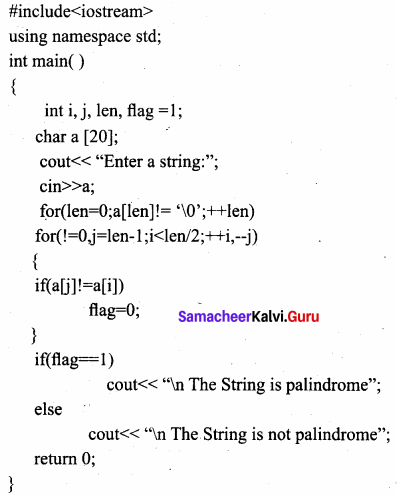Output:
Enter a string : madam The String is palindrome

Question 3.
Write about returning structures from functions.
Answer:
A structure can be passed to a function through its object. Therefore, passing a structure to a function or passing a structure object to a function is the same because structure object represents the structure. Like a normal variable, structure variable(structure object) can be passed by value or by references / addresses. Similar to built-in data types, structures also can be returned from a function.

Question 4.
What is the output of the following program?
Answer: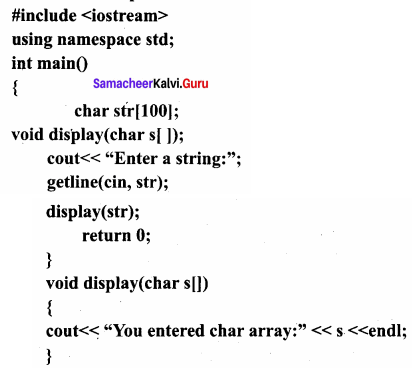Output:
Enter a string: welcome to C++ programming
You entered char array: welcome to C++ programming

Question 5.
What is global object?
Answer:
Objects declared along with structure definition are called global objects.Question 6.
What is the condition of structure assignments?
Answer:
Structure assignment is possible only if both structure variables/objects are same type.

Question 7.
Why structures are usually passed by reference method?
Answer:
Structures are usually passed by reference method because it saves the memory space and executes faster.

Question 8.
What is the size of the following highlighted variable in terms of byte if it is compiled in dev C++?
Answer:
struct A{ float f; char ch;long double d;};
struct B{Aa; int arr;}b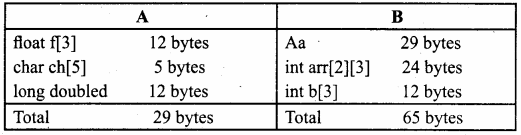Question 9.
Is the following snippet is fully correct. If not identify the error.
Answer:
struct suml{ int n1,n2;}s1;
struct sum2{int n1,n2}s2;
cin >> s1.n1 >> s1.n2; s2=s1;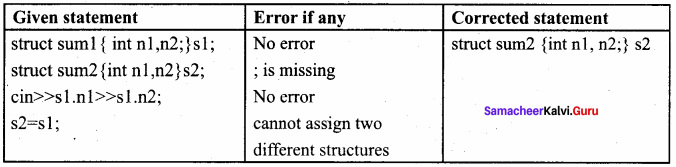Question 10.
Differentiate array and structure.
Answer:
Array:

• An array is a collection of variables of same data type.
• Array data are accessed using index.
• Array allocates static memory
• Array element access takes lesser time.

Structure:

• A structure is a collection of variables of different data type.
• Structure elements are accessed using operator.
• Structures allocate dynamic memory.
• Structure elements takes more time.Question 11.
What are the different ways to initialize the structure members?
Answer:
Values can be assigned to structure elements similar to assigning values to variables.
Example
balu.rollno= “702016”;
balu.age= 18;
balu.weight= 48.5;
Also, values can be assigned directly as similar to assigning values to Arrays.
balu={702016, 18, 48.5};

Question 12.
What is wrong with the following C++ declarations?
Answer:
A. struct point (double x, y )
B. struct point { double x, double y };
C. struct point { double x; double y }
D. struct point { double x; double y;};
E. struct point { double x; double y;}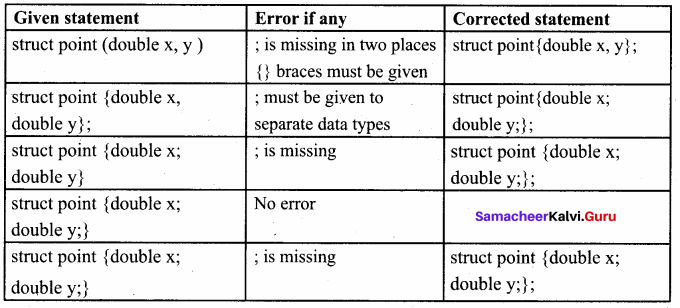PART – 3
III. Short Answers

Question 1.
Write about initialization of 2 – D array.
Answer:
The array can be initialized in more than one way at the time of 2-D array declaration.
For example
int matrix = {
{10,20,30},// Initializes row 0
{40,50,60},// Initializes row 1
{70,80,90},// Initializes row 2
{100,110,120}// Initializes row 3
}; .
int matrix = {10, 20, 30, 40, 50, 60, 70, 80, 90, 100, 110, 120};
Array’s row size is optional but column size is compulsory.

Question 2.
What is row major order and column major order?
Answer:
In row – major order, all the elements are stored row by row in continuous memory locations, that is, all the elements in first row, then in the second row and so on. The memory representation of row major order is as shown below;
Row major order: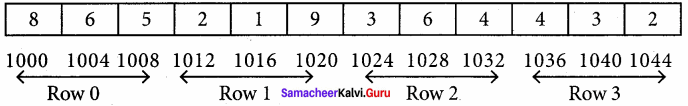Column major order: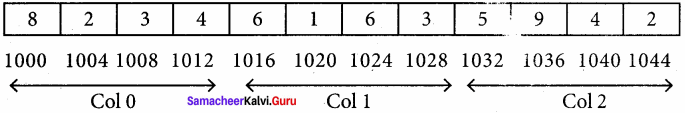Question 3.
What is array of strings?
Answer:
An array of strings is a two – dimensional character array. The size of the first index (rows) denotes the number of strings and the size of the second index (columns) denotes the maximum length of each string. Usually, array of strings are declared in such a way to accommodate the null character at the end of each string. For example, the 2 – D array has the declaration: char Name;
In the above declaration, the 2 – D array has two indices which refer to the row size and column size, that is 6 refers to the number of rows and 10 refers to the number of columns.Question 4.
Write a C++ program to access array elements using pointer with sample output.
Answer:
#include
using namespace std;
int main()
{

int data ;
cout << “Enter elements:”;
for(int i = 0; i <5; ++i) cin >> data [i];
cout << “You entered:”;
for (int i = 0; i < 5;++i)
cout << endl << *(data +i);

}
return 0;
Output:
Enter elements:
1
2
3
5
4
You entered:
1
2
3
5
3

Question 5.
Write a program to assign data to members of a structure variable and display the contents.
Answer:
C++ Program that assigns data to members of a structure variable and displays the contents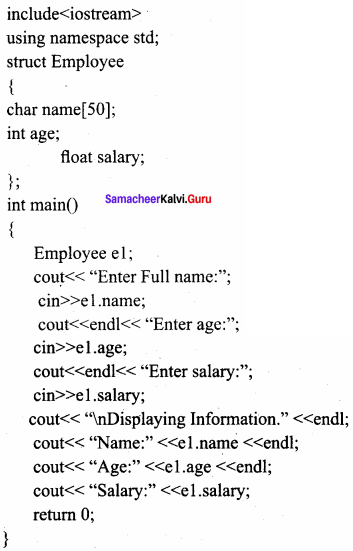Output:
Enter Full name:
Ezhil Enter age:
27
Enter salary:
40000.00
Displaying Information.
Name: Ezhil
Age: 27
Salary: 40000.00Question 6.
What is called nested structure? Give example.
Answer:
The structure declared within another structure is called a nested structure. Nested structures act as members of another structure and the members of the child structure can be accessed as parent structure name. Child structure name. Member name, struct dob
{
int date;
char month;
int year;
} ;
Values can be assigned to this structure as follows.
dob = {25, “DEC”, 2017}

Question 7.
Rewrite the following program after removing the syntactical error(s),if any. Underline each, correction.
Answer: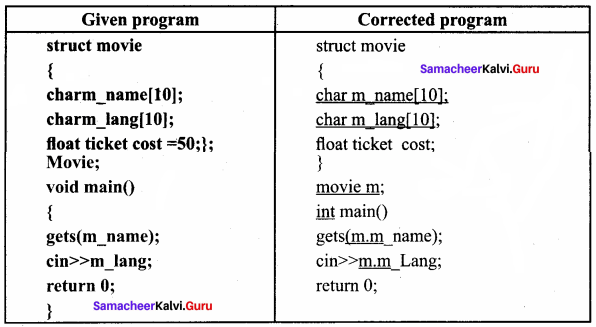Question 8.
What is the difference among the following two programs?
Answer:
(a) #include
struct point { double x; double y;};
int main() {
struct point test;
test.x = .25; testy = .75;
cout << test.x << test.y;
return 0;
}

(b) #include
struct { double x; double y;} Point;
int main(void) {
Point test={.25,.75};
return 0;
}

(a) Output

1. 0.250.75
2. Named structure point is created.

(b)

1. No output: Error
2. Anonymous structure point is created.

Question 9.
For the following structure definition write the user defined function to accept data through keyboard.
struct date{ int dd,mm,yy};
struct item {int item id;char name;float price;date datemanif;}
Answer:
void accept (item & i)
{

cout << “\n Enter the Item id”; cin >> i.id;
cout << “\n Enter the item name:”; cin >> i.name;
cout << “\n Enter the item price:”; cin >> i.price;
cout << “\n Enter the item manufacturing day:”; cin >> i.date.dd;
cout << “\n Enter the item manufacturing month:”; cin >> i.date.mm;
cout << “\n Enter the item manufacturing year:”; cin >> i.date.yy;

}Question 10.
Write a user defined function to return the structure after accepting value through keyboard. The structure definition is as follows: struct Item{int item no;float price;};
Answer:
item accept (item i)
{

cout << “\n Enter the Item No:”; cin >> i.no;
cout << “\n Enter the Item Price:”; cin >> i.price;
return i;

}

PART – 4
IV. Explain in Detail

Question 1.
Write C++ program to find transpose of a matrix.
Answer:
C++ Program to find transpose of a matrix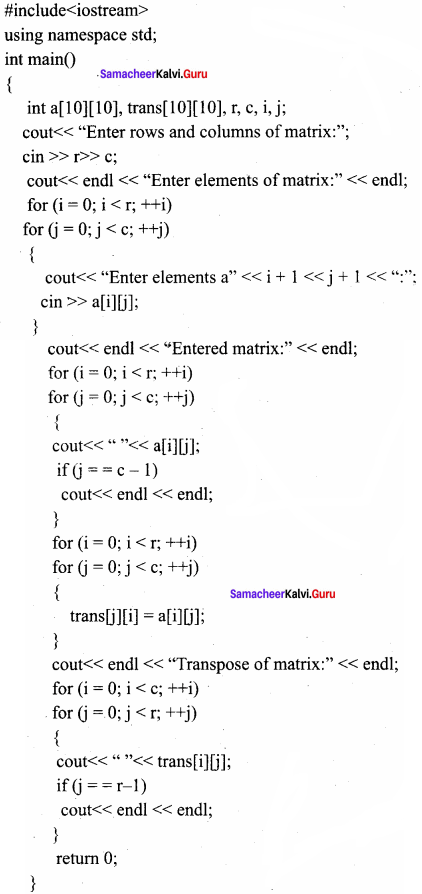Output:
Enter rows and columns of matrix:
2 3
Enter elements of matrix
Enter elements of a1 1 = 1
Enter elements of a1 2 = 2
Enter elements of a1 3 = 9
Enter elements of a2 1 = 0
Enter elements of a2 2 = 4
Enter elements of a2 3 = 7
Entered matrix:
1 2 9 0 4 7
Transpose of matrix:
10 2 4 9 7

Question 2.
Write C++ program to sort words in dictionary order.
C++ Program to find transpose of a matrix
Answer: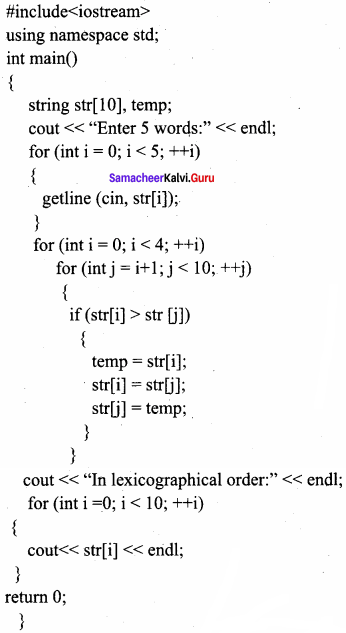Output:
Enter 10 words:
Kalai
Artqueen
Visalatchi
Jaya
Dhanush
In lexicographical order:
Artqueen
Dhanush
Jaya
Kalai
Visalatchi

Question 3.
Explain memory representation of 2 – D array.
Answer:
Normally, the two – dimensional array can be viewed as a matrix. The conceptual view of a 2 – D array is shown below:
int A;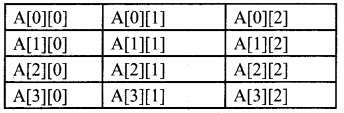In the above example, the 2 – D array name A has 4 rows and 3 columns.
Like one – dimensional, the 2 – D array elements are stored in continuous memory. There are two types of 2 – D array memory representations. They are:

1. Row – Major order
2. Column – Major order

For example
intA = {

{8,6,5},
{2,1,9},
{3,6,4},
{4,3,2},

Row major: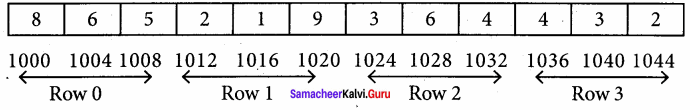Column major order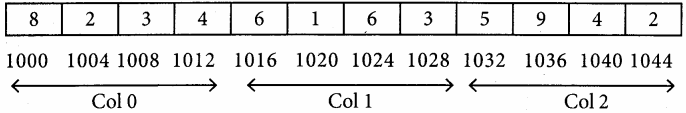Question 4.
Let an organisation have three employees. If we want to read and print all their details, write a C++program for the following structure definition
struct Employee
{

char name ;
int age;
float salary;

};
Answer: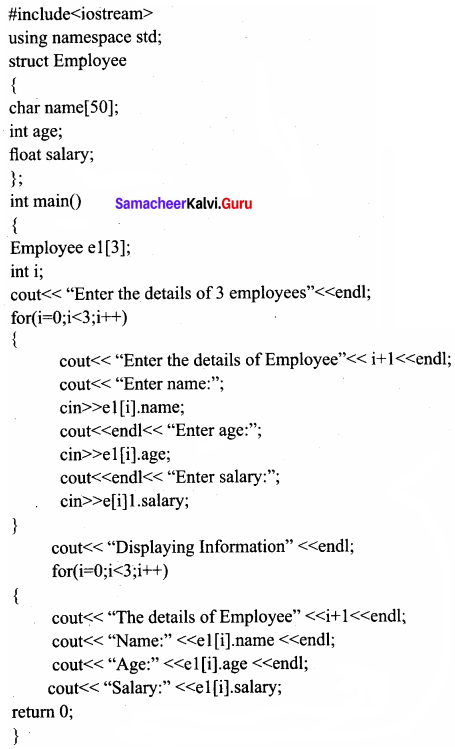Output:
Enter the details of 3 employees:
Enter the details of Employee 1
Enter name:
Lilly

Enter age:
42

Enter salary:
40000.00

Enter the details of Employee 2
Enter name:
Aster

Enter age:
38

Enter salary:
60000.00

Enter the details of Employee 3
Enter name:
Jasmine

Enter age:
45

Enter salary:
80000.00

Displaying Information:
The details of Employee 1
Name : Lilly
Age : 42
Salary : 40000.00

The details of Employee 2
Name : Aster
Age : 38
Salary : 60000.00

The details of Employee 3
Name : Jasmine
Age : 45
Salary : 80000.00

Question 5.
Explain returning structures from functions with an example.
Answer:
A structure can be passed to a function through its object. Therefore, passing a structure to a function or passing a structure object to a function is the same because structure object represents the structure. Like a normal variable, structure variable (structure object) can be passed by value or by references / addresses. Similar to built – in data types, structures also can be returned from a function.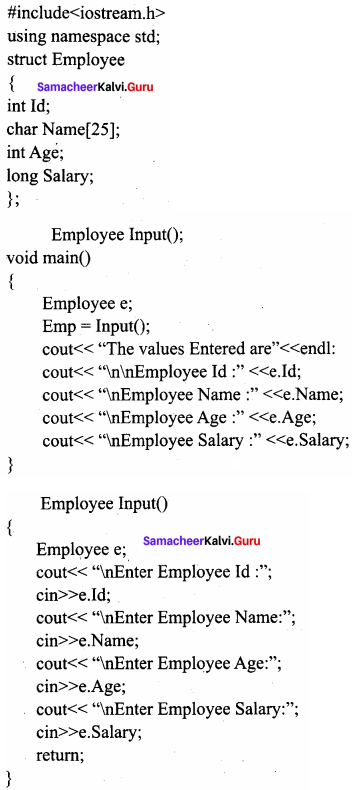Output:
Enter Employee Id : 10
Enter Employee Name : Ajay
Enter Employee Age : 25
Enter Employee Salary : 15000
Employee Id : 10
Employee Name : Ajay
Employee Age : 25
Employee Salary : 15000

Question 6.
Write output of the following program.
Answer: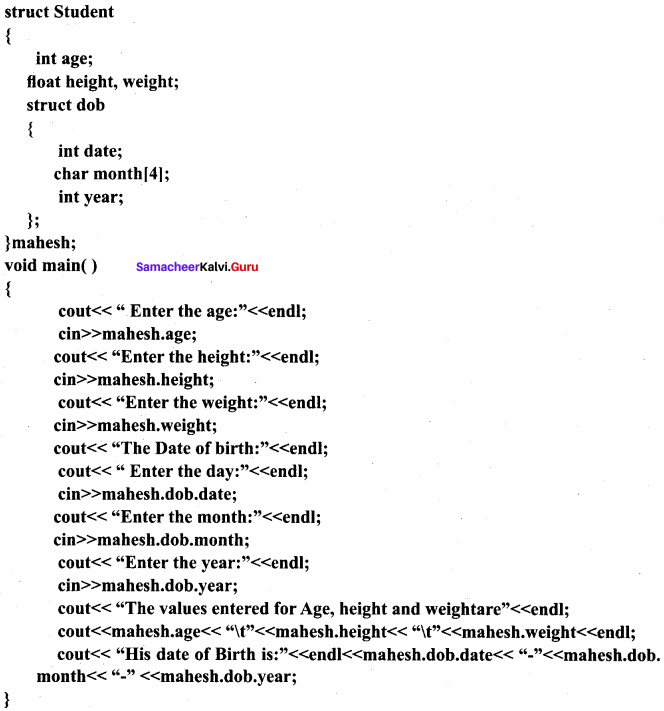Output:
Enter the age:
18
Enter the height:
160.5
Enter the weight:
46.5
The Date of birth Enter the day:
25
Enter the month:
NOV
Enter the year:
2017
The values entered for Age, height and weight are
18 160.5 46.5
His date of Birth is:
25 – NOV – 2017